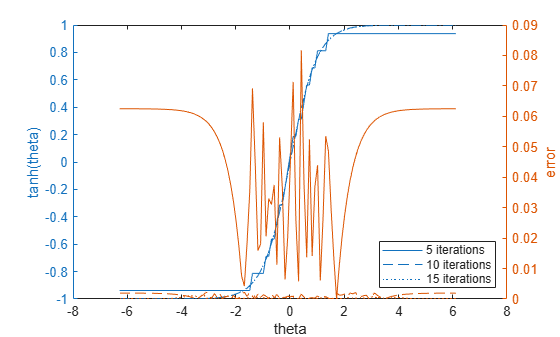# cordictanh

CORDIC-based hyperbolic tangent

## Syntax

``T = cordictanh(theta)``
``T = cordictanh(theta, niters)``

## Description

example

````T = cordictanh(theta)` returns the hyperbolic tangent of `theta`.```

example

````T = cordictanh(theta, niters)` returns the hyperbolic tangent of theta by performing `niters` iterations of the CORDIC algorithm.```

## Examples

collapse all

Find the hyperbolic tangent of `fi` object `theta` using a CORDIC implementation with the default number of iterations.

```theta = fi(-2*pi:.1:2*pi-.1); T_cordic = cordictanh(theta);```

Plot the hyperbolic tangent of `theta` using the `tanh` function and its CORDIC approximation.

```T = tanh(double(theta)); plot(theta, T_cordic); hold on; plot(theta, T); legend('CORDIC approximation of tanh', 'tanh'); xlabel('theta'); ylabel('tanh(theta)');```Compute the difference between the results of the `cordictanh` function and the `tanh` function.

```figure; err = abs(T - double(T_cordic)); plot(theta, err); xlabel('theta'); ylabel('error');```Find the hyperbolic tangent of `fi` object `theta` using a CORDIC implementation and specify the number of iterations the CORDIC kernel should perform. Plot the CORDIC approximation of the hyperbolic tangent of theta with varying numbers of iterations.

```theta = fi(-2*pi:.1:2*pi-.1); for niters = 5:10:25 T_cordic = cordictanh(theta, niters); plot(theta, T_cordic); hold on; end xlabel('theta'); ylabel('tanh(theta)'); legend('5 iterations', '15 iterations', '25 iterations','Location','southeast');```## Input Arguments

collapse all

Angle values in radians specified as a scalar, vector, matrix, or N-dimensional array.

Data Types: `single` | `double` | `int8` | `int16` | `int32` | `int64` | `uint8` | `uint16` | `uint32` | `uint64` | `fi`

The number of iterations that the CORDIC algorithm performs, specified as a positive, integer-valued scalar. If you do not specify `niters`, the algorithm uses a default value. For fixed-point inputs, the default value of `niters` is one less than the word length of the input array, `theta`. For double-precision inputs, the default value of `niters` is 52. For single-precision inputs, the default value is 23.

Data Types: `single` | `double` | `int8` | `int16` | `int32` | `int64` | `uint8` | `uint16` | `uint32` | `uint64` | `fi`

## Output Arguments

collapse all

`T` is the CORDIC-based approximation of the hyperbolic tangent of `theta`. When the input to the function is floating point, the output data type is the same as the input data type. When the input is fixed point, the output has the same word length as the input, and a fraction length equal to the `WordLength``2`.